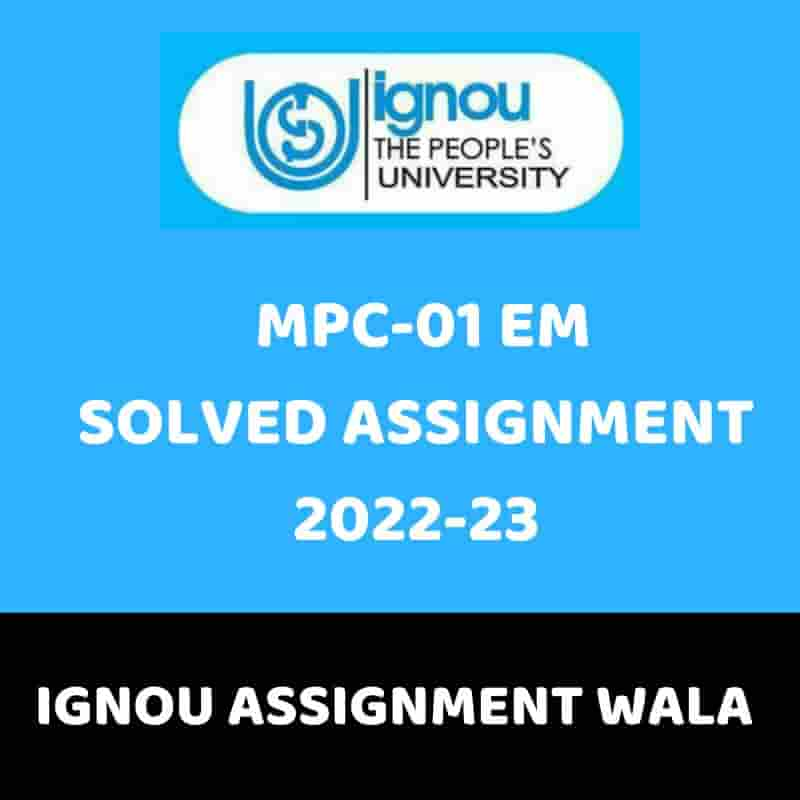## IGNOU MPC-01 SOLVED ASSIGNMENT 2022-23

Cognitive Psychology, Learning and Memory (MPC-001)
TUTOR MARKED ASSIGNMENT
(TMA)

SECTION – A

Answer the following questions in 1000 words each. 3 x 15 = 45 marks
1. Discuss the structure and functions of language.
2. Critically discuss Das, Naglieri and Kirby’s PASS theory.
3. Explain the barriers to problem solving.

SECTION – B

Answer the following questions in 400 words each. 5 x 5 = 25 marks
4. Describe the levels of processing model by Craik and Lockhart.
5. Explain the factors affecting problem solving.
6. Discuss Guilford’s model of intelligence.
7. Define creativity. Describe the stages of creativity.
8. Explain the key issues in the study of cognitive psychology.

SECTION – C

Answer the following questions in 50 words each. 10 x 3 = 30 marks
9. Seven primary factors given by Thurstone
10. Aspects of creativity
11. Heuristics
12. Long term memory
13. Role of hippocampus in memory
14. Four principles of information processing
15. Neuroscience and cognitive psychology
16. Problem space hypothesis
17. Means-ends analysis
18. Types of problems